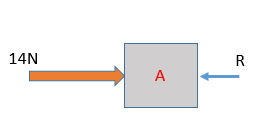Type Here to Get Search Results !

# Numerical based on blocks on smooth surface

## Numericals based on blocks on smooth surface

Question 1:

Find the acceleration of 6kg,4kg,10kg block ? It is assumed that all susfaces are friction less and smooth.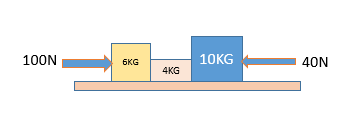(a) 18 m/s², 12 m/s², 30 m/s²
(b) 18 m/s², 30 m/s², 12 m/s²
(c) 12 m/s², 18 m/s², 30 m/s²
(d) None of these
Answer:(a) 18 m/s², 12 m/s², 30 m/s²

Solution: Total mass of system :M= 6+4+10=20Kg
Net force on the system :F= 100-40=60N
Acceleration of system: 60/20=3 m/s²
Force on 6kg block =6*3=18N
Force on 4kg block =4*3=12N
Force on 6kg block =10*3=30N

Question 2:

Find the acceleration of each block?It is assumed that both block are palced on friction less surface.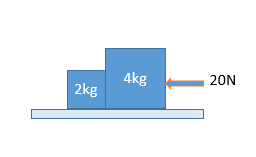(a) 10 m/s²
(b) 10/3 m/s²
(c) 3 m/s²
(d) None of these

Solution: Total mass of system :M= 2+4=6Kg
Net force on the system :F=20N
Acceleration of system: 20/6=10/3 m/s²

Solution:
Case1:
Total mass of system :M= 1+2=Kg
Net force on the system :F=6N
Acceleration of system: 6/3=2 m/s²
Let contact force between the block= N1
6-N1=1*2
NI=6-2=4N

Case2:
Total mass of system :M= 1+2=Kg
Net force on the system :F=6N
Acceleration of system: 6/3=2 m/s²
Let contact force between the block= N2
6-N2=2*2
N2=6-4=2N
Ratio of reaction forces
N1:N2=4/2=2:1

Question 4:

Two blocks of masses m and M are connected by an inextensible light string . What would be tension in string when force F act on block of mass M as shown figure below:

(a) (mF)/(m+M)
(b) (MF)cosθ/(m+M)
(c) (mF)cosθ/(m+M)
(d) None of these

Solution:

Question 5:

Two blocks A and B wach mass m each are paced on smooth horizontal surface.Two forces F and 2F are applied on the block A nad B respectively as shown in figure below.The block A doenot slide over on block B at contact surface.What would be the normal reaction N between the blocks.
(a) N=3F/2
(b) N=F/3
(c) N=3F
(d) None of these

Solution:

Question 6:

Rigid blocks are kept in contact on a frictionless surface as shown in figure .What would be contact forces between A&B and contact force between B&C.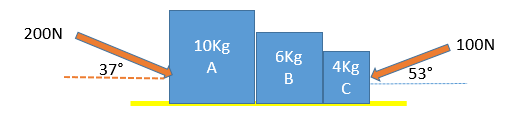(a) 110N,80N
(b) 120N,80N
(c) 130N,80N
(d) None of these

Solution:
Fnet= (200cos37°)-(100cos53°)
Fnet= (200*4/5)-(100*3/5)
Fnet=160-60 =100N
Total mass of system=10+6+4=20Kg
a= 100/20=5m/s²
Calculation of contact forces between A & B:
FBD of block A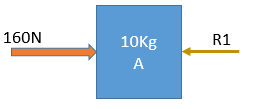160-R1=10*5
R1=160-50=110N
Calculation of contact forces between B & C:
FBD of block B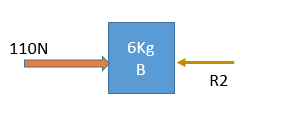110-R2=6*5
R2=110-30=80N

Question 7:

Find the contact force between block 4Kg and 2KG mass?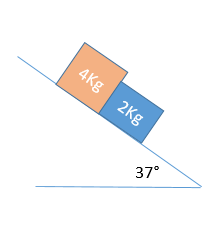(a) 0N
(b) 4N
(c) 7N
(d) None of these

Solution: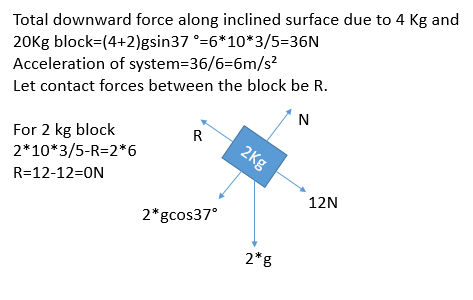Question 8:

Rigid blocks are kept in contact on a frictionless surface as shown in figure .What would be contact forces between A&B and contact force between B&C.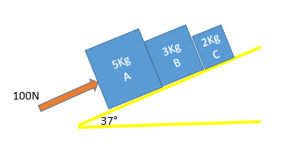(a) 40N,20N
(b) 50N,20N
(c) 60N,20N
(d) None of these

Solution: Fnet=100-(5+3+2)gsin37°
Fnet=100-10*10*3/5
Fnet=100-60 =40N
Total mass of system=5+3+2=10Kg
a= 40/10=4m/s²
Calculation of contact forces between A & B: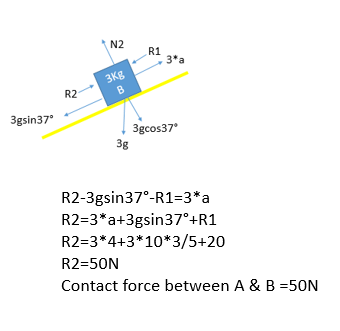Calculation of contact forces between B & C: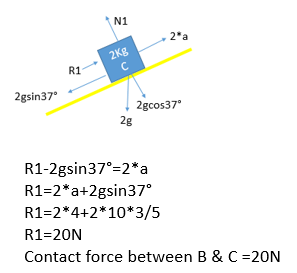Question 9:

Three blocks A,B and C masses 4Kg,2Kg and 1Kg respectively are in contact on a smooth surface as shown fig. below A force of 14 N is applied on 4Kg block What would be contact force between A & B.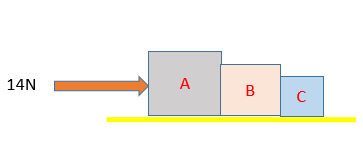(a) 4N
(b) 5N
(c) 6N
(d) None of these